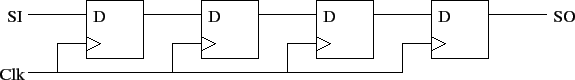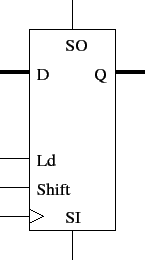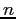# Shift Registers

Tom Kelliher, CS 240

Apr. 23, 2012

Registers

### Outline

1. Shift registers defined.

3. VHDL

Counters

# Shift registers defined

1. Why is a parallel register parallel?

2. So, we would expect a shift (serial) register to look like:SO = SI four clocks later.

Using 2-1 muxes, how would you modify this to incorporate a shift control signal?

3. Parallel register with shift:D and Q are buses.

1. Suppose you have two serial bit streams, A and B. Design a serial adder using one one bit full adder and one D flip-flop.

If A and B arebits, the output can be how many bits?

2. Suppose A and B are shifted in on a single bit line. Is it possible for us to do the addition? (One shift register needed.)

3. What does a left shift by one do to the value of an unsigned number?

Use this to design a sequential circuit which takes A as serial input and outputs 3A.

# VHDL for Serial Registers

Parallel load, shift left or right, hold.

```-- Parallel load shift register.  Shift left or right.
-- Mode bits:
--    00: hold
--    10: shift left (toward msb)
--    11: shift right (toward lsb)
--
-- msi: most significant shift in.
-- lsi: least significant shift in.

library ieee;
use ieee.std_logic_1164.all;

entity shift_reg is

port (
d            : in  std_logic_vector (31 downto 0);
mode         : in  std_logic_vector (1 downto 0);
clk, reset_n : in  std_logic;
msi, lsi     : in  std_logic;
q            : out std_logic_vector (31 downto 0));

end shift_reg;

architecture behavioral of shift_reg is

signal state : std_logic_vector (31 downto 0);

begin  -- behavioral

q <= state;                           -- Update output.

state_register: process (clk, reset_n)
begin  -- process state_register
if reset_n = '0' then               -- asynchronous reset (active low)
state <= X"00000000";
elsif clk'event and clk = '1' then  -- rising clock edge
if mode = "00" then               -- Hold.
state <= state;
elsif mode = "01" then            -- Load.
state <= d;
elsif mode = "10" then            -- Shift left.
state <= state (30 downto 0) & lsi;
else                              -- Shift right.
state <= msi & state (31 downto 1);
end if;
end if;
end process state_register;

end behavioral;
```

Thomas P. Kelliher 2012-04-24
Tom Kelliher